# A5 1-D and 2-D Convolutional Neural Networks in Pytorch¶

• A5.4: Changed structure of CNN2D.__init__ by having it call make_cnn_and_fc_layers function. It is this function that CNN1D must override., not the __init__ constructor.
• A5.3: Added two missing statements in CNN2D that initialize layeri to 0 and increment it by 1
• A5.2: added an exception to CNN2D.__init__ code that provides a helpful message if you specify an impossible configuration for convolutional layers. Repeat this exception code in your CNN1D.__init__ function.
• A5.1: small edit in CNN2D to allow empty list for n_hiddens_per_fc_layer.

In this assignment, you will experiment with the given convolutional neural network for 2-dimensional input samples (images), in class CNN2D, by applying it to the MNIST data. You will also define a new class for handling 1-dimensional input samples, called CNN1D, that extends CNN2D.

In :
import numpy as np
import torch
import pandas

import matplotlib.pyplot as plt


## CNN2D class¶

Here is a definition of CNN2D.

In :
import numpy as np
import torch

class CNN2D(torch.nn.Module):

def __init__(self, n_inputs, n_hiddens_per_conv_layer, n_hiddens_per_fc_layer, n_outputs,
patch_size_per_conv_layer, stride_per_conv_layer, activation_function='tanh', device='cpu'):

super().__init__()

self.device = device

n_conv_layers = len(n_hiddens_per_conv_layer)
if (len(patch_size_per_conv_layer) != n_conv_layers
or len(stride_per_conv_layer) != n_conv_layers):
raise Exception('The lengths of n_hiddens_per_conv_layer, patch_size_per_conv_layer, and stride_per_conv_layer must be equal.')

self.activation_function = torch.tanh if activation_function == 'tanh' else torch.relu

self.make_conv_and_fc_layers(n_inputs, n_hiddens_per_conv_layer, n_hiddens_per_fc_layer, n_outputs,
patch_size_per_conv_layer, stride_per_conv_layer)

self.Xmeans = None
self.to(self.device)

def make_conv_and_fc_layers(self, n_inputs, n_hiddens_per_conv_layer, n_hiddens_per_fc_layer, n_outputs,
patch_size_per_conv_layer, stride_per_conv_layer):
# Create all convolutional layers
# First argument to first Conv2d is number of channels for each pixel.
# Just 1 for our grayscale images.
n_in = 1
input_hw = int(np.sqrt(n_inputs))  # original input image height (=width because image assumed square)
self.conv_layers = torch.nn.ModuleList()
layeri = 0
for nh, patch_size, stride in zip(n_hiddens_per_conv_layer,
patch_size_per_conv_layer,
stride_per_conv_layer):
self.conv_layers.append(torch.nn.Conv2d(n_in, nh, kernel_size=patch_size, stride=stride))
conv_layer_output_hw = (input_hw - patch_size) // stride + 1
if conv_layer_output_hw <= 0:
raise Exception(f'''For conv layer {layeri}, input_hw of {input_hw} is less than patch_size {patch_size}.
Try reducing the patch_size for this layer or for the previous layer.''')
input_hw = conv_layer_output_hw  # for next trip through this loop
n_in = nh
layeri += 1

# Create all fully connected layers.  First must determine number of inputs to first
# fully-connected layer that results from flattening the images coming out of the last
# convolutional layer.
n_in = input_hw ** 2 * n_in
self.fc_layers = torch.nn.ModuleList()
for nh in n_hiddens_per_fc_layer:
self.fc_layers.append(torch.nn.Linear(n_in, nh))
n_in = nh
self.fc_layers.append(torch.nn.Linear(n_in, n_outputs))

def forward_all_outputs(self, X):
n_samples = X.shape
Ys = [X]
for conv_layer in self.conv_layers:
Ys.append(self.activation_function(conv_layer(Ys[-1])))

flattened_input = Ys[-1].reshape(n_samples, -1)

for layeri, fc_layer in enumerate(self.fc_layers[:-1]):
if layeri == 0:
Ys.append(self.activation_function(fc_layer(flattened_input)))
else:
Ys.append(self.activation_function(fc_layer(Ys[-1])))

if len(self.fc_layers) == 1:
# only the output layer
Ys.append(self.fc_layers[-1](flattened_input))
else:
Ys.append(self.fc_layers[-1](Ys[-1]))

return Ys

def forward(self, X):
Ys = self.forward_all_outputs(X)
return Ys[-1]

def train(self, X, T, batch_size, n_epochs, learning_rate, method='sgd', verbose=True):
'''X and T must be numpy arrays'''

self.classes = np.unique(T)
T = np.arange(len(self.classes))[np.where(T.reshape(-1, 1) == self.classes)]

# Set data matrices to torch.tensors
X = torch.from_numpy(X).float().to(self.device)
T = torch.from_numpy(T).long().to(self.device)  # required for classification in pytorch

# Setup standardization parameters
if self.Xmeans is None:
self.Xmeans = X.mean(axis=0)
self.Xstds = X.std(axis=0)
self.Xstds[self.Xstds == 0] = 1  # So we don't divide by zero when standardizing

# Standardize X
X = (X - self.Xmeans) / self.Xstds

if method == 'sgd':
optimizer = torch.optim.SGD(self.parameters(), lr=learning_rate, momentum=0.9)
else:

CELoss = torch.nn.CrossEntropyLoss(reduction='mean')
self.error_trace = []

for epoch in range(n_epochs):

num_batches = X.shape // batch_size
loss_sum = 0

for k in range(num_batches):
start = k * batch_size
end = (k + 1) * batch_size
X_batch = X[start:end, ...]
T_batch = T[start:end, ...]

Y = self.forward(X_batch)

loss = CELoss(Y, T_batch)
loss.backward()

# Update parameters
optimizer.step()

loss_sum += loss

self.error_trace.append(loss_sum / num_batches)

if verbose and (epoch + 1) % (max(1, n_epochs // 10)) == 0:
print(f'{method}: Epoch {epoch + 1} Loss {self.error_trace[-1]:.3f}')

return self

def softmax(self, Y):
'''Apply to final layer weighted sum outputs'''
# Trick to avoid overflow
maxY = torch.max(Y, axis=1).reshape((-1, 1))
expY = torch.exp(Y - maxY)
denom = torch.sum(expY, axis=1).reshape((-1, 1))
Y = expY / denom
return Y

def use(self, X):
# Set input matrix to torch.tensors
X = torch.from_numpy(X).float().to(self.device)
# Standardize X
X = (X - self.Xmeans) / self.Xstds
# Calculate output of net for all samples in X
Y = self.forward(X)
# Convert output to class probabilities
probs = self.softmax(Y)
# For each sample pick highest probability and translate that to class labels
classes = self.classes[torch.argmax(probs, axis=1).cpu().numpy()].reshape(-1, 1)
return classes, probs.detach().cpu().numpy()


### CNN2D on MNIST Digits¶

We will use a bunch (50,000) images of hand drawn digits from this deeplearning.net site. Download mnist.pkl.gz.

This pickle file includes data already partitioned into training, validation, and test sets. To read it into python, use the following steps

In :
import pickle
import gzip

with gzip.open('mnist.pkl.gz', 'rb') as f:
train_set, valid_set, test_set = pickle.load(f, encoding='latin1')

Xtrain = train_set
Ttrain = train_set.reshape(-1, 1)

Xval = valid_set
Tval = valid_set.reshape(-1, 1)

Xtest = test_set
Ttest = test_set.reshape(-1, 1)

Xtrain = Xtrain.reshape(-1, 1, 28, 28)
Xtest = Xtest.reshape(-1, 1, 28, 28)

print(Xtrain.shape, Ttrain.shape,  Xval.shape, Tval.shape,  Xtest.shape, Ttest.shape)

(50000, 1, 28, 28) (50000, 1) (10000, 784) (10000, 1) (10000, 1, 28, 28) (10000, 1)

In :
device = 'cpu'
if torch.cuda.is_available():
y_or_n = input('Would you like to run on the GPU? (y or n): ')
if y_or_n == 'y' or y_or_n == 'yes':
device = torch.device('cuda:0' if torch.cuda.is_available() else 'cpu')
print('Running on', device)

Would you like to run on the GPU? (y or n): y
Running on cuda:0

In :
len(np.unique(Ttrain))

Out:
10
In :
n_hiddens_per_conv_layer = [10, 10]
patch_size_per_conv_layer = [10, 5]
stride_per_conv_layer=[4, 2]
n_hiddens_per_fc_layer = 

cnnet = CNN2D(28 * 28, n_hiddens_per_conv_layer, n_hiddens_per_fc_layer, len(np.unique(Ttrain)),
patch_size_per_conv_layer, stride_per_conv_layer, device=device)

n_epochs = 20
batch_size = 500
learning_rate = 0.01

cnnet.train(Xtrain, Ttrain, batch_size, n_epochs, learning_rate, method='adam')

plt.plot(cnnet.error_trace, label='Pytorch')
plt.title('MNIST')

adam: Epoch 2 Loss 0.451

Out:
Text(0.5, 1.0, 'MNIST')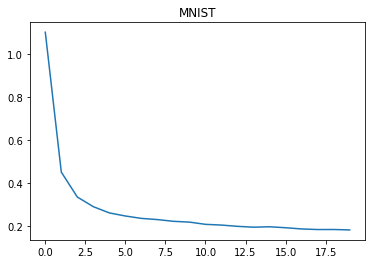In :
def confusion_matrix(Y_classes, T):
class_names = np.unique(T)
table = []
for true_class in class_names:
row = []
for Y_class in class_names:
row.append(100 * np.mean(Y_classes[T == true_class] == Y_class))
table.append(row)
conf_matrix = pandas.DataFrame(table, index=class_names, columns=class_names)
return conf_matrix

In :
Classes, _ = cnnet.use(Xtest)
perc_correct = 100 * np.mean(Classes == Ttest)
print(f'Test accuracy in percent correct: {perc_correct:.2f}')
confusion_matrix(Classes, Ttest)

Test accuracy in percent correct: 93.75

Out:
0 1 2 3 4 5 6 7 8 9
0 95.918367 0.000000 0.510204 0.408163 0.102041 0.612245 1.734694 0.408163 0.102041 0.204082
1 0.000000 97.797357 0.616740 0.176211 0.088106 0.000000 0.528634 0.088106 0.616740 0.088106
2 0.581395 0.581395 94.573643 1.162791 0.096899 0.484496 0.290698 0.872093 1.356589 0.000000
3 0.099010 0.000000 2.277228 91.683168 0.000000 1.485149 0.198020 1.089109 3.168317 0.000000
4 0.101833 0.000000 0.000000 0.101833 94.399185 0.000000 1.120163 0.712831 0.712831 2.851324
5 0.224215 0.000000 0.448430 1.569507 0.672646 92.040359 1.008969 0.336323 3.699552 0.000000
6 0.835073 0.521921 0.521921 0.000000 0.521921 1.043841 96.555324 0.000000 0.000000 0.000000
7 0.194553 0.291829 2.431907 0.875486 0.389105 0.194553 0.000000 94.163424 0.583658 0.875486
8 0.205339 1.129363 0.821355 3.182752 0.102669 0.924025 0.410678 0.821355 91.683778 0.718686
9 0.594648 0.495540 0.099108 0.891972 5.351833 0.396432 0.099108 2.378593 1.585728 88.107037

### Experiments¶

To explore the effects of different CNN structures, show results for the following steps. For each use the same number of epochs, batch size, and learning rate as used above.

1. Compare test accuracy of CNN2D nets with one, two and three convolutional layers, each with 10 units and patch sizes of 5 and strides of 2.
2. Using the best number of convolutional layers found in Step 1, compare the test accuracies of CNN2d nets with zero, one, and two fully-connected layers each with 10 hidden units.

Combine the results of each of your runs and display them in a pandas.Dataframe that includes the network structure and percent correct on train and test sets. Discuss your results, and describe the network structure and training parameters that produced the best test results.

## CNN1D¶

Complete the following code cell to define CNN1D. The only change from CNN2D that is required is in the constructor. Complete these steps.

1. Copy the __init__ function from CNN2D.
2. For each convolutional layer, create a torch.nn.Conv1d object instead of a torch.nn.Conv2d object.
3. Modify the statement input_hw = int(np.sqrt(n_inputs)) appropriately.
4. Modify the statement n_in = input_hw ** 2 * n_in appropriately.
In [ ]:
class CNN1D(CNN2D):

def make_conv_and_fc_layers(self, n_inputs, n_hiddens_per_conv_layer, n_hiddens_per_fc_layer, n_outputs,
patch_size_per_conv_layer, stride_per_conv_layer):

. . .


### Toy Data to Test CNN1D¶

Here is some toy data to test your CNN1D definition. Each sample is now 1-dimensional. Let's make vectors of two kinds, ones with square pulses and ones with triangular pulses, at random locations and random durations. Both kinds will be 100 values, with zeros between the waves long.

In :
def make_square_pulse():
sample = np.zeros(100)
for i in range(np.random.randint(1, 5)):  # making from 1 to 4 pulses
start = np.random.randint(0, 80)
width = np.random.randint(5, 20)
sample[start:start + width] = 1
return sample

In :
make_square_pulse()

Out:
array([0., 0., 0., 0., 0., 0., 0., 0., 0., 0., 0., 0., 0., 0., 0., 0., 0.,
0., 0., 0., 0., 0., 0., 0., 0., 0., 0., 0., 0., 0., 0., 0., 0., 0.,
0., 0., 0., 0., 0., 0., 0., 0., 0., 0., 0., 0., 0., 0., 0., 0., 0.,
0., 0., 1., 1., 1., 1., 1., 1., 1., 0., 0., 0., 1., 1., 1., 1., 1.,
0., 0., 0., 0., 0., 0., 0., 0., 0., 0., 0., 0., 0., 0., 0., 0., 0.,
0., 0., 0., 0., 0., 0., 0., 0., 0., 0., 0., 0., 0., 0., 0.])
In :
plt.plot(make_square_pulse());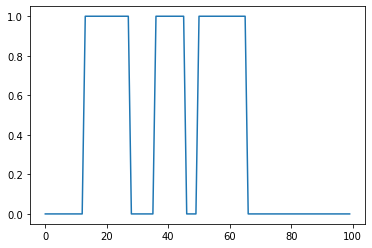In :
def make_triangular_pulse():
sample = np.zeros(100)
for i in range(np.random.randint(1, 5)):  # making from 1 to 4 pulses
start = np.random.randint(0, 80)
width = np.random.randint(5, 20)
if width % 2 == 1:
width += 1  # if odd, make it even
sample[start:start + width // 2] = np.linspace(0, 1, width // 2)
sample[start + width // 2:start + width] = np.linspace(1, 0, width // 2)
return sample

In :
plt.plot(make_triangular_pulse());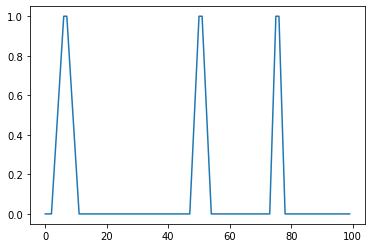In :
n_each = 500
Xtrain = np.array([make_square_pulse() for i in range(n_each)] +
[make_triangular_pulse() for i in range(n_each)])
Ttrain = np.array(['square'] * n_each + ['triangular'] * n_each).reshape(-1, 1)
n_each = 500
Xtest = np.array([make_square_pulse() for i in range(n_each)] +
[make_triangular_pulse() for i in range(n_each)])
Ttest = np.array(['square'] * n_each + ['triangular'] * n_each).reshape(-1, 1)
Xtrain.shape, Ttrain.shape, Xtest.shape, Ttest.shape

Out:
((1000, 100), (1000, 1), (1000, 100), (1000, 1))
In :
np.newaxis == None

Out:
True
In :
Xtrain = Xtrain[:, None, :]
Xtrain.shape

Out:
(1000, 1, 100)
In :
Xtrain = Xtrain.reshape(Xtrain.shape, 1, -1)
Xtest = Xtest.reshape(Xtest.shape, 1, -1)
Xtrain.shape, Xtest.shape

Out:
((1000, 1, 100), (1000, 1, 100))
In :
cnnet1 = CNN1D(100, [10, 5], [5, 5], 2, [10, 4], [1, 2], device=device)

n_epochs = 100
batch_size = 10
learning_rate = 0.001

cnnet1.train(Xtrain, Ttrain, batch_size, n_epochs, learning_rate, method='adam')

plt.plot(cnnet1.error_trace, label='Pytorch')
plt.title('Pulses')

adam: Epoch 10 Loss 0.682

Out:
Text(0.5, 1.0, 'Pulses')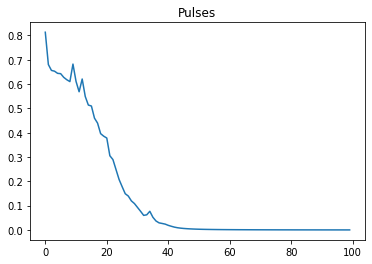In :
Classes, _ = cnnet1.use(Xtest)
perc_correct = 100 * np.mean(Classes == Ttest)
print(f'Test accuracy in percent correct: {perc_correct:.2f}')
confusion_matrix(Classes, Ttest)

Test accuracy in percent correct: 99.10

Out:
square triangular
square 98.2 1.8
triangular 0.0 100.0
In :
W = list(cnnet1.children()).weight.data.cpu()  # in case running on GPU
plt.plot(W[:, 0, :].T);
W[:, 0, :].T.shape

Out:
torch.Size([10, 10])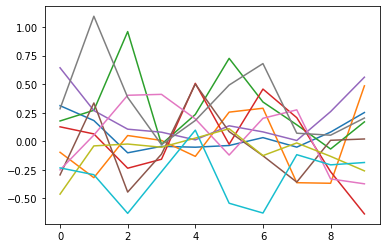### Experiments on ECG Data¶

An electrocardiogram, or ECG, is a record in time of a voltage generated by the heart. It can be used to diagnose abnormalities in the heart.

Public datasets containing ECG traces are available, such as the Non-Invasive Fetal ECG Arrhythmia Database site. The data files there are in a standard waveform-database (WFDB) format. As is often the case for most standard data formats you run in to, a python package exists for reading this data, called wfdb that you can install using conda.

This data set includes ECG from normal patients and from ones with arrythmias, with data file names like ARR_01.dat and NR_01.dat, respectively. We have already downloaded these files, read them in using the wfdb package and collected them into segments of 2000 voltages. The sample rate for this data is 1000 Hz, so 2000 voltages spans 2 seconds. Download this data set from ecg.npy

Now, our job for our CNN1D is to classify each 2000 sample segment into the classes normal or arrythmia.

After you have downloaded ecg.npy, you can load it and plot a few samples.

In :
ecg = np.load('ecg.npy')
arr = ecg['arrythmia']
norm = ecg['normal']
arr.shape, norm.shape

Out:
((2429, 2000), (3634, 2000))
In :
plt.figure(figsize=(15, 15))

plt.subplot(2, 2, 1)
plt.plot(arr)
plt.legend(('Arrythmia',))
plt.subplot(2, 2, 2)
plt.plot(arr)
plt.legend(('Arrythmia',))

plt.subplot(2, 2, 3)
plt.plot(norm)
plt.legend(('Normal',))
plt.subplot(2, 2, 4)
plt.plot(norm)
plt.legend(('Normal',));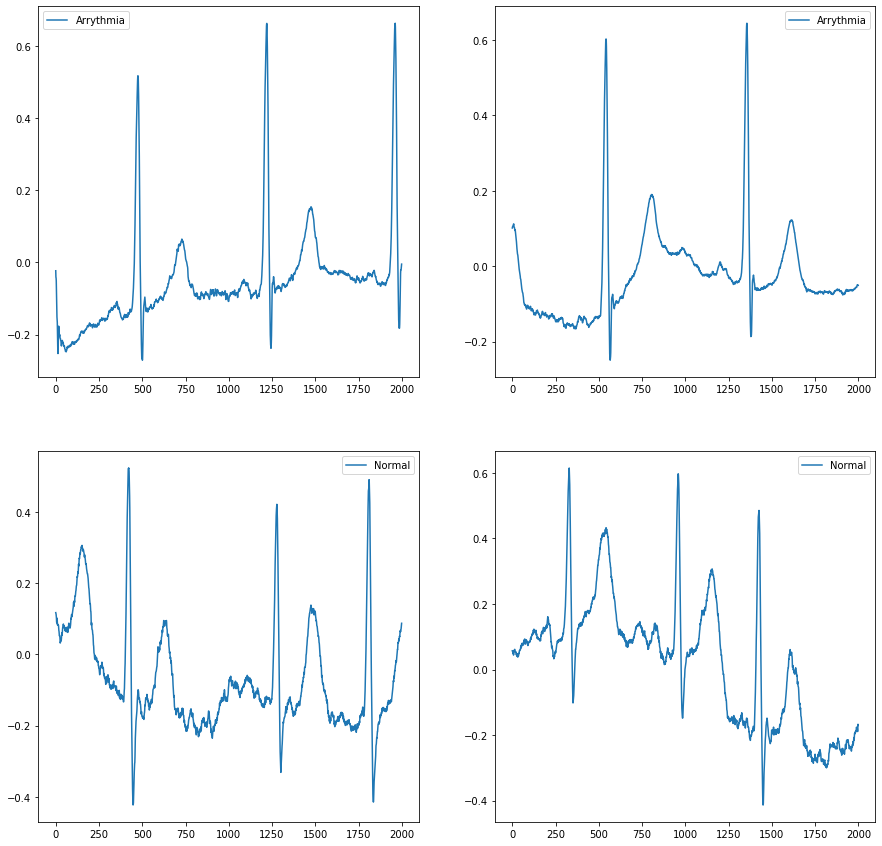Now, let's stack the arr and norm samples together, create class labels for each sample, randomly rearrange them, and divide into train and test sets.

In :
X = np.vstack((arr, norm))
X = X.reshape(X.shape, 1, -1)
T = np.hstack((['arr'] * arr.shape, ['nr'] * norm.shape)).reshape(-1, 1)
n_samples = X.shape
rows = np.arange(n_samples)
np.random.shuffle(rows)
n_train = int(n_samples * 0.8)
Xtrain = X[rows[:n_train], ...]
Ttrain = T[rows[:n_train], ...]
Xtest = X[rows[n_train:], ...]
Ttest = T[rows[n_train:], ...]

Xtrain.shape, Ttrain.shape, Xtest.shape, Ttest.shape

Out:
((4850, 1, 2000), (4850, 1), (1213, 1, 2000), (1213, 1))
In :
Ttrain

Out:
array([['nr'],
['arr'],
['nr'],
...,
['nr'],
['nr'],
['nr']], dtype='<U3')

Okay, ready to train. Create a CNN1D network with a statements like this.

In :
cnn1d = CNN1D(Xtrain.shape[-1], [5, 10], [10, 10], 2, [100, 20], [20, 5], device=device)


Now, experiment with at least ten different network structures, patch sizes and strides and compare them with the percent accuracy on test data. Combine the results of each of your runs and display them in a pandas.Dataframe that includes the network structure and percent correct on train and test sets. Discuss your results, and describe the network structure and training parameters that produced the best test results.

In :
%run -i A5grader.py

Testing

xs = np.arange(100)
n_each = 500
n_samples = n_each * 2
X = np.array([np.sin(xs / 2) + np.random.normal(0, 1, size=100) for i in range(n_each)] +
[np.sin(xs / 3) + np.random.normal(0, 1, size=100) for i in range(n_each)])
X = X[:, np.newaxis, :]
T = np.array( * n_each +  * n_each).reshape(-1, 1)
rows = np.arange(n_samples)
np.random.shuffle(rows)
X = X[rows, ...]
T = T[rows, ...]
n_train = int(n_samples * 0.8)
Xtrain = X[:n_train, ...]
Ttrain = T[:n_train, :]
Xtest = X[n_train:, ...]
Ttest = T[n_train:, :]

cnn1d = CNN1D(100, [5, 5], , 2, [10, 5], [1, 2])
cnn1d.train(Xtrain, Ttrain, 10, 20, 0.01, method='adam')

perc_train = 100 * np.mean(cnn1d.use(Xtrain) == Ttrain)
perc_test = 100 * np.mean(cnn1d.use(Xtest) == Ttest)

--- 40/40 points.  perc_train is correctly 100%.

--- 40/40 points.  perc_test is correctly 100%.

======================================================================
A5 Execution Grade is 80 / 80
======================================================================

__ / 10 Based on results of experiments with MNIST data.

__ / 10 Based on results of experiments with ECG data.

======================================================================
A5 FINAL GRADE is  _  / 100
======================================================================

Extra Credit:

Earn up to 3 extra credit points on this assignment by doing any or all of the following experiments.

1. Compare your results on the MNIST data by using relu versus tanh activation functions. Show and
discuss the results.

2. Compare your results on the MNIST data using adam versus sgd. Show and discuss the results.

3. Download another image data set, apply your CNN2D class to this data and discuss the results.

A5 EXTRA CREDIT is 0 / 3


## Extra Credit¶

Earn up to 3 extra credit points on this assignment by doing any or all of the following experiments.

1. Compare your results on the MNIST data by using relu versus tanh activation functions. Show and discuss the results.
2. Compare your results on the MNIST data using adam versus sgd. Show and discuss the results.
3. Download another image data set, apply your CNN2D class to this data and discuss the results.